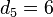# Determination of character table of alternating group:A5

This page describes the process used for the determination of specific information related to a particular group. The information type is character table and the group is alternating group:A5.
View all pages that describe how to determine character table of particular groups | View all specific information about alternating group:A5
This page is incomplete. At present, it only includes the determination of the degrees of irreducible representations, not the entire character table.

This page describes various ways to determine the character table of alternating group:A5. The idea is to determine as much as possible wit has little knowledge of the representation theory of these groups as we can manage, therefore making the discussion suitable for people who know only basic facts about the groups and basic facts of linear representation theory. For a detailed discussion of the character theory, see linear representation theory of alternating group:A5.

## Finding the degrees of irreducible representations using number-theoretic constraints

Final answer: The degrees of irreducible representations are 1, 3, 3, 4, 5.

The following facts are known in general:

1. Sum of squares of degrees of irreducible representations equals order of group: In this case, it tells us that the sum of the degrees of irreducible representations is 60, the order of the group.
2. Number of irreducible representations equals number of conjugacy classes: In this case, it tells us that there are 5 irreducible representations, because there are 5 conjugacy classes.
3. Degree of irreducible representation divides order of group: In this case, it tells us that the degrees of irreducible representations all divide 60, the order of the group.
4. Number of one-dimensional representations equals order of abelianization: In this case, the group is a perfect group (in fact, it is a simple non-abelian group -- see A5 is simple) so there is only one one-dimensional representation.
5. Order of inner automorphism group bounds square of degree of irreducible representation

(2) tells us that there are five irreducible representations. Denote their (positive integer) degrees, in non-decreasing order, as$d_1 \le d_2 \le \dots \le d_5$. (1) tells us that$d_1^2 + d_2^2 + d_3^2 + d_4^2 + d_5^2 = 60$. Note that$d_5 \le 7$ because$8^2 = 64 > 60$. Moreover, (3) tells us that all the degrees must divide 60, this eliminates the case$d_5 = 7$. There exists a trivial representation, so$d_1 = 1$ and$1 \le d_2 \le d_3 \le d_4 \le d_5$. We thus have:$d_2^2 + d_3^2 + d_4^2 + d_5^2 = 59$

with$1 \le d_2 \le d_3 \le d_4 \le d_5 \le 6$. Note that we can use (4) to show that$2 \le d_2$, but we will be able to narrow down to a unique choice of degrees of irreducible representations even without this information.

### Case$d_5 = 6$

In this case, we obtain:$d_2^2 + d_3^2 + d_4^2 = 23$

However, 23 cannot be expressed as a sum of three squares. So this case is eliminated.

### Case$d_5 = 5$

In this case, we obtain:$d_2^2 + d_3^2 + d_4^2 = 34$

Consider the subcases:

•$d_4 = 5$: This gives$d_2^2 + d_3^2 = 9$, but 9 is not the sum of two squares of positive integers. So this case is ruled out.
•$d_4 = 4$: This gives$d_2^2 + d_3^2 = 18$, giving the solution$d_2 = d_3 = 3$. We thus get$d_1 = 1, d_2 = 3, d_3 = 3, d_4 = 4, d_5 = 5$.
•$d_4 \le 3$: This is not possible, because$34 = d_2^2 + d_3^2 + d_4^2 \le 3d_4^2$, so$d_4^2 \ge 34/3$.

### Case$d_5 \le 4$

In this case, we obtain:$d_2^2 + d_3^2 + d_4^2 = 43$

with$d_4 \le 4$. By size considerations,$d_4 = 4$ is forced, and$d_3 = 4$ is also forced. But this leaves$d_2^2 = 11$, which does not give an integer value of$d_2$.